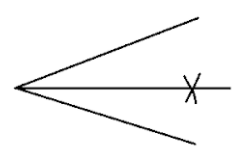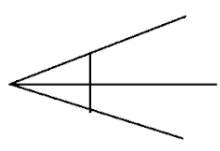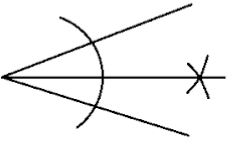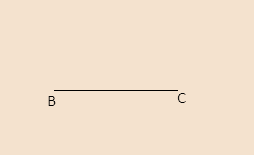Filters
Latest Questions
CBSE
Mathematics
Construction of angles
Draw a right angle and construct its bisector.
CBSE
Mathematics
Construction of angles
The steps of construction of an \$\angle AOB={{45}^{\circ }}\$ is given in jumbled order below:
1. Place compass on intersection point.
2. Place ruler on start point and where arc intersects perpendicular line.
3. Adjust compass width to reach start point.
4. Construct a perpendicular line.
5. Draw a 45-degree line.
6. Draw an arc that intersects a perpendicular line.
The third step in process is:
(a) 2
(b) 3
(c) 4
(d) 5
CBSE
Mathematics
Construction of angles
Draw an angle of measure \${127^o}\$ and construct its bisector.
CBSE
Mathematics
Construction of angles
Draw an angle of \[{{50}^{\circ }}\] with the help of a protractor. Draw a ray bisecting this angle.
CBSE
Mathematics
Construction of angles
Construct an angle of \[75^\circ \] and bisect it.

CBSE
Mathematics
Construction of angles
Construct an angle of \[75^\circ \] and bisect it.

CBSE
Mathematics
Construction of angles
Which diagram below shows a correct mathematical construction using only a compass and a straightedge to bisect an angle?
(a)(b)(c)(d) None of these

CBSE
Mathematics
Construction of angles
How to draw \${{300}^{\circ }}\$ in standard position and find one positive and one negative angle that is coterminal to a given angle.

CBSE
Mathematics
Construction of angles
Construct the following angles using set squares: 75 degree

CBSE
Mathematics
Construction of angles
Construct the angle of \$105{}^\circ \$ only with the help of a ruler and compass.
CBSE
Mathematics
Construction of angles
BC is given. Construct \$\angle ABC\$, \$\angle PBC\$ and \$\angle QBC\$ such that m \$\angle ABC = {60^\circ }\$, m \$\angle PBC = {30^ \circ }\$ and m \$\angle QBC = {15^\circ }\$ with the help of a pair of compass and straight edge. Write the steps of construction.CBSE
Mathematics
Construction of angles
Draw an angle of measure\[{147^ \circ }\]and construct its bisector.

Prev
1
2
3